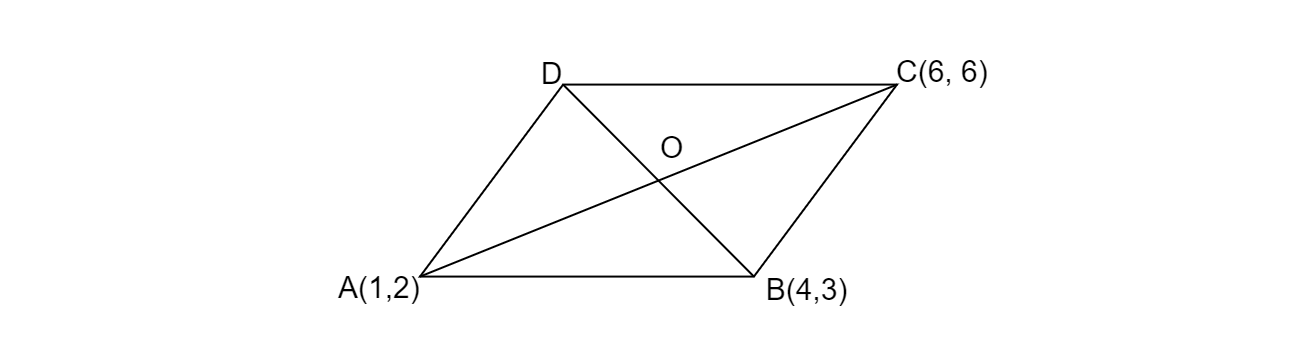# If $A( 1,2) ,B( 4,3)$ and $C( 6,\ 6)$ are the three vertices of a parallelogram $ABCD$, find the coordinates of fourth vertices D.

Given: A parallelogram ABCD with the vertices $A(1,\ 2), B(4,\ 3)$ and $C(6,\ 6)$
To do: To find out the coordinates of the fourth vertices D.
Solution:
Here ABCD is a parallelogram, AC and BD are its diagonals to each other and intersects at the point O.Point is the mid-point of AC and BD.
If O is the mid-point of AC.
$\Rightarrow O=\left(\frac{1+6}{2} ,\ \frac{2+6}{2}\right)$
$\therefore O=\left(\frac{7}{2} ,4\right)$
O is the mid-point of BD also.
Let $( x,\ y)$ is point D.
$\therefore O=\left(\frac{( x+4)}{2} ,\frac{( y+3)}{2}\right)$
But we know O$=\left(\frac{7}{2} ,4\right)$
$\therefore \left(\frac{7}{2} ,4\right) =\left(\frac{( x+4)}{2} ,\frac{( y+3)}{2}\right)$
$\Rightarrow \ \ \frac{( x+4)}{2} =\frac{7}{2} \ and\ \frac{( y+3)}{2} =4$
$\Rightarrow x+4=7$ and $y+3=8$
$\Rightarrow$
$x=7-4=3$ and $y=8-3=5$
thus $D=( 3,\ 5)$
The co-ordinates of fourth vertex $D=( 3,\ 5)$

Updated on: 10-Oct-2022

64 Views